# RNN-Time-series-Anomaly-Detection

RNN based Time-series Anomaly detector model implemented in Pytorch.

This is an implementation of RNN based time-series anomaly detector, which consists of two-stage strategy of time-series prediction and anomaly score calculation.

## Requirements

• Ubuntu 16.04+ (Errors reported on Windows 10. see issue. Suggesstions are welcomed.)
• Python 3.5+
• Pytorch 0.4.0+
• Numpy
• Matplotlib
• Scikit-learn

## Dataset

1. NYC taxi passenger count

2. Electrocardiograms (ECGs)

• The ECG dataset containing a single anomaly corresponding to a pre-ventricular contraction

3. 2D gesture (video surveilance)

• X Y coordinate of hand gesture in a video

4. Respiration

• A patients respiration (measured by thorax extension, sampling rate 10Hz)

5. Space shuttle

• Space Shuttle Marotta Valve time-series

6. Power demand

• One years power demand at a Dutch research facility

The Time-series 2~6 are provided by E. Keogh et al. in "HOT SAX: Efficiently Finding the Most Unusual Time Series Subsequence." In The Fifth IEEE International Conference on Data Mining. (2005) , dataset

## RNN-based Multi-Step Prediction Model

0. Architecture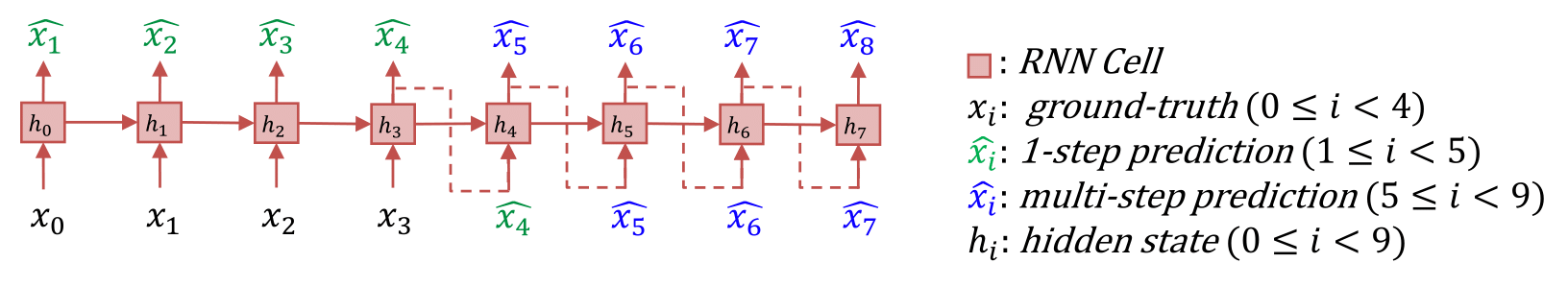When the value of x_i is known from i=0 to i=t, the model recursively predicts the value of x_i from i=t+1 to i=T. In this figure, t=3, T=8. We first train this model with a trainset which contains no anomalies, then we use the trained model to detect anomalies in a testset, where anomalies are included.

1. How to train this model

Recursive multi-step prediction using RNNs is a rather difficult problem. As the prediction progresses, the prediction errors are accumulated and the predictions rapidly become inaccurate. To solve this problem, we need a model that is robust to input noise.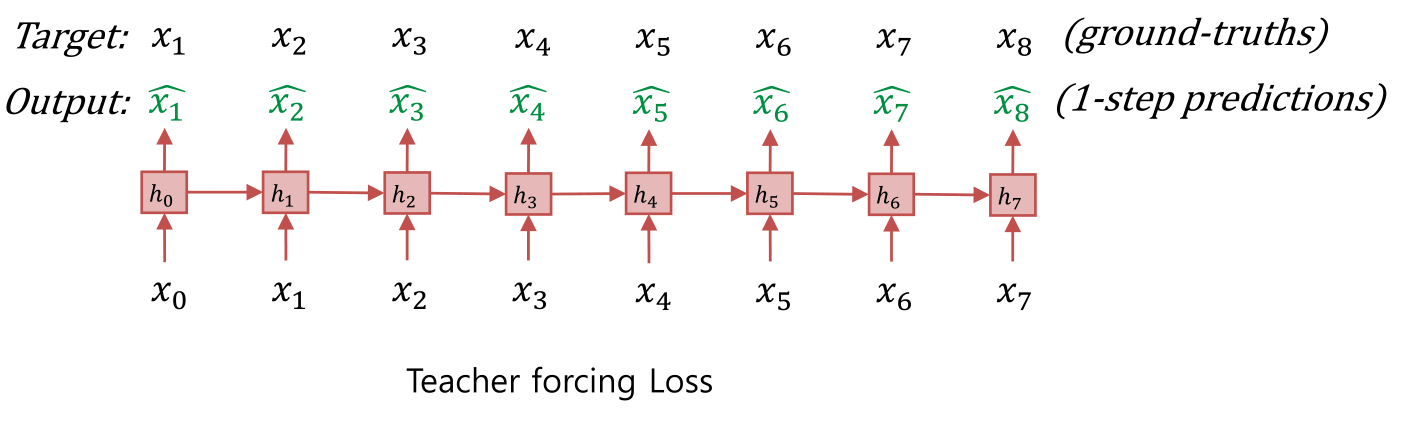TODO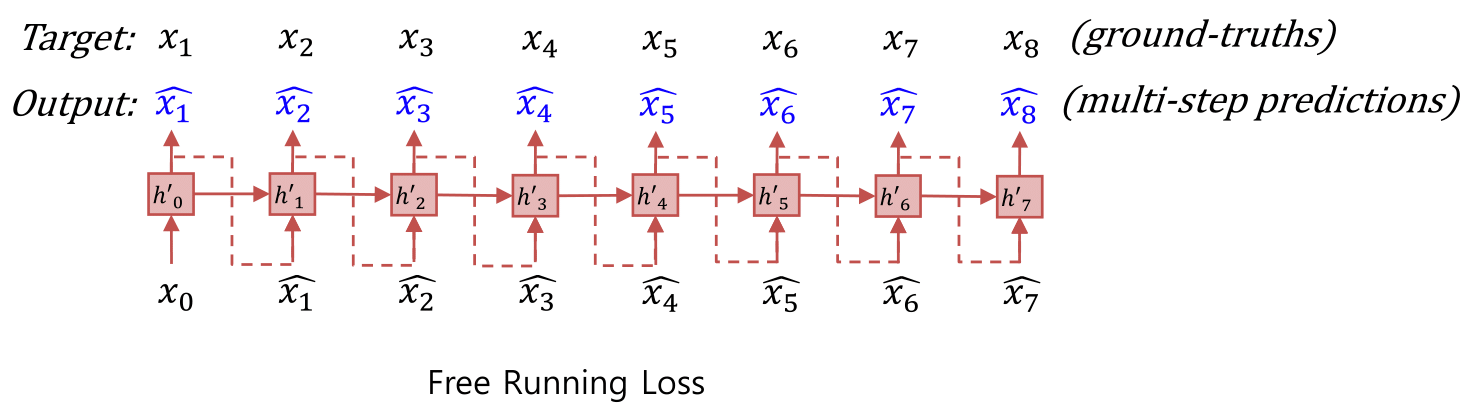TODO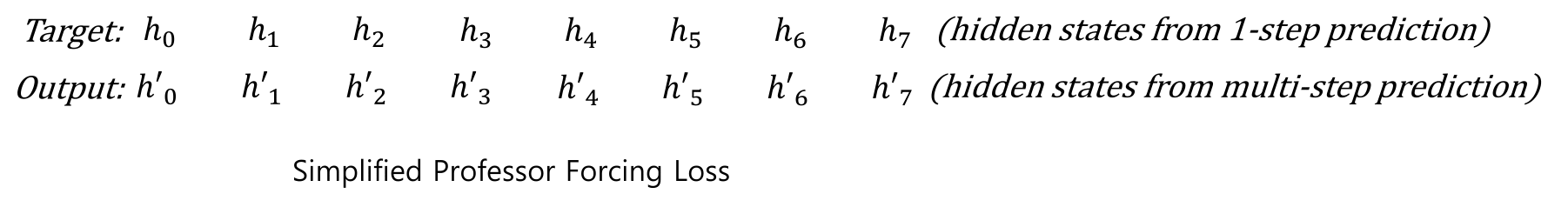TODO

TODO

## Example of usage

0. Download the dataset: Download the five kinds of multivariate time-series dataset (ecg, gesture,power_demand, respiration, space_shuttle), and Label all the abnormality points in the dataset.

``````    python 0_download_dataset.py
``````

1. Time-series prediction: Train and save RNN based time-series prediction model on a single time-series trainset

``````    python 1_train_predictor.py --data ecg --filename chfdb_chf14_45590.pkl
python 1_train_predictor.py --data nyc_taxi --filename nyc_taxi.pkl
``````

Train multiple models using bash script

``````    ./1_train_predictor_all.sh
``````

2. Anomaly detection: Fit multivariate gaussian distribution and calculate anomaly scores on a single time-series testset

``````    python 2_anomaly_detection.py --data ecg --filename chfdb_chf14_45590.pkl --prediction_window 10
python 2_anomaly_detection.py --data nyc_taxi --filename nyc_taxi.pkl --prediction_window 10
``````

Test multiple models using bash script

``````    ./2_anomaly_detection_all.sh
``````

## Result

1. Time-series prediction: Predictions from the stacked RNN model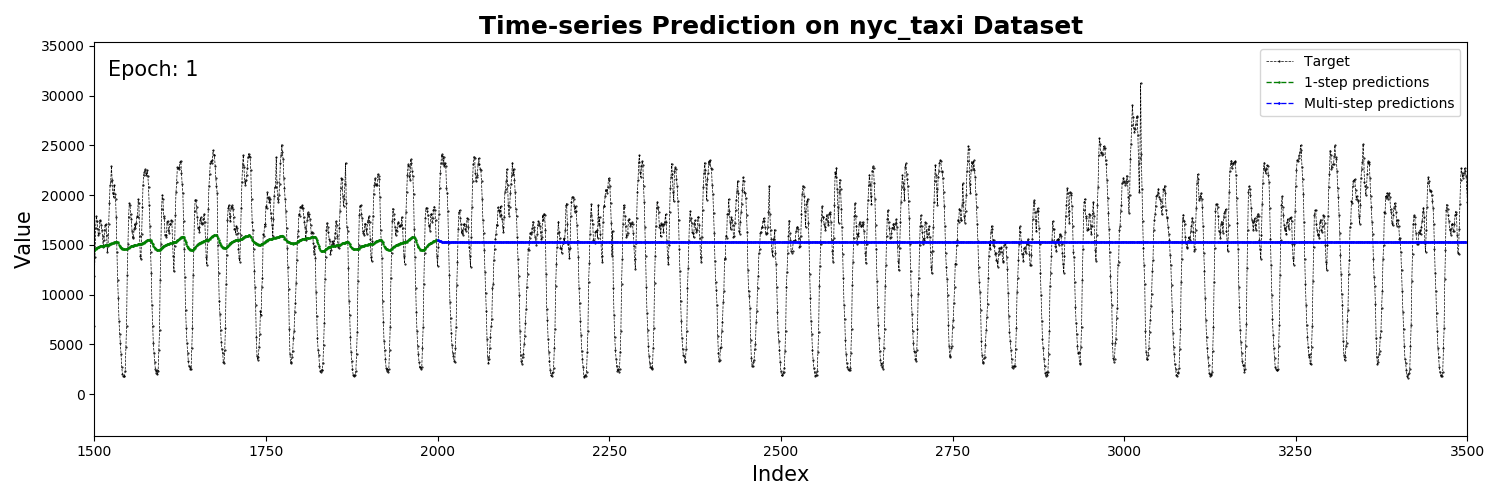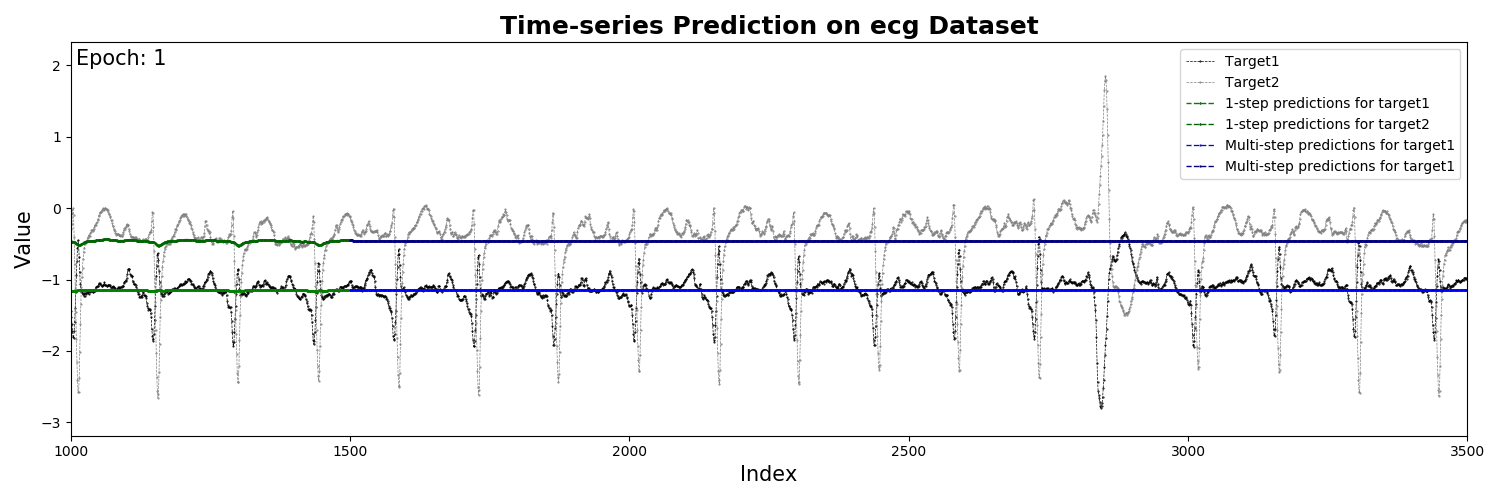2. Anomaly detection:

Anomaly scores from the Multivariate Gaussian Distribution model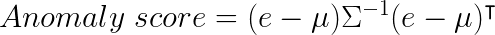• NYC taxi passenger count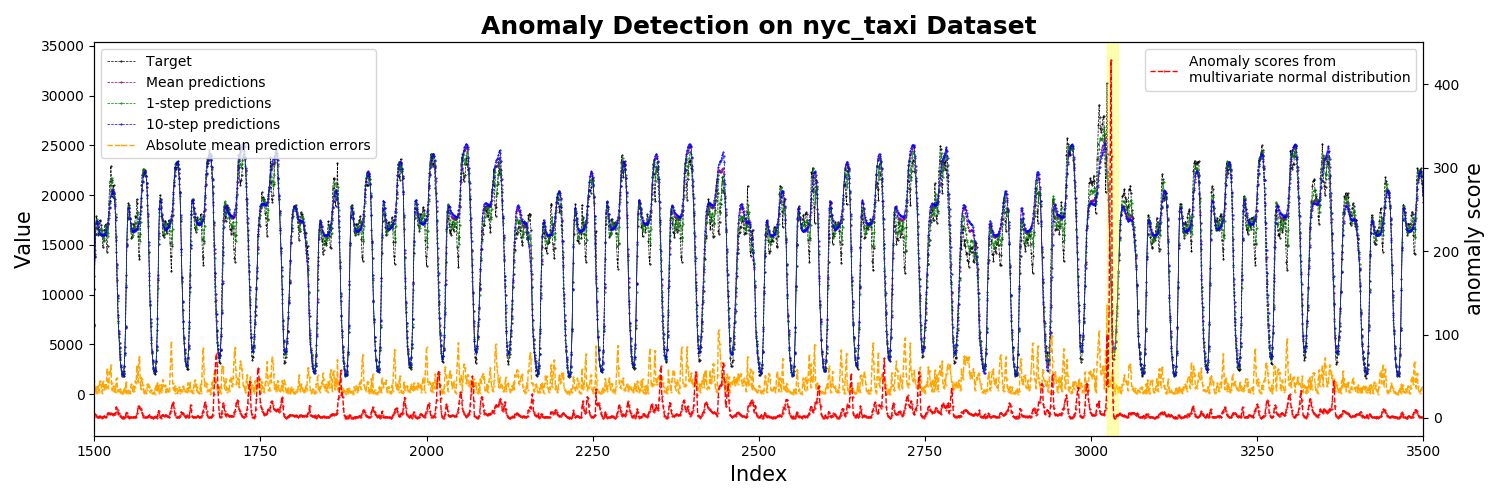• Electrocardiograms (ECGs) (filename: chfdb_chf14_45590)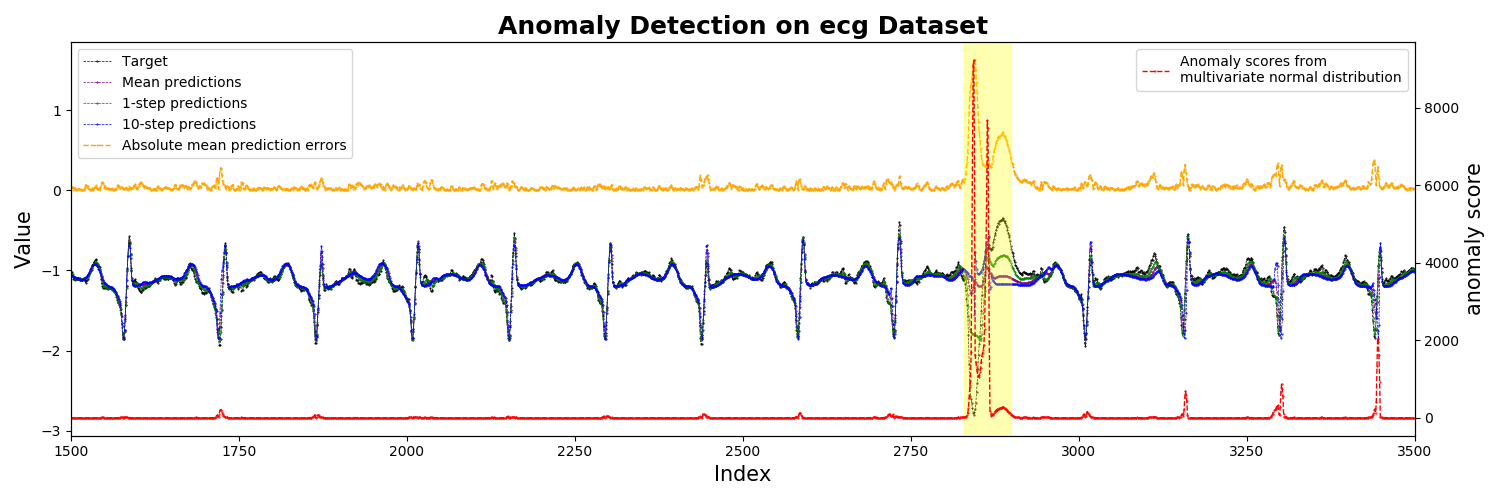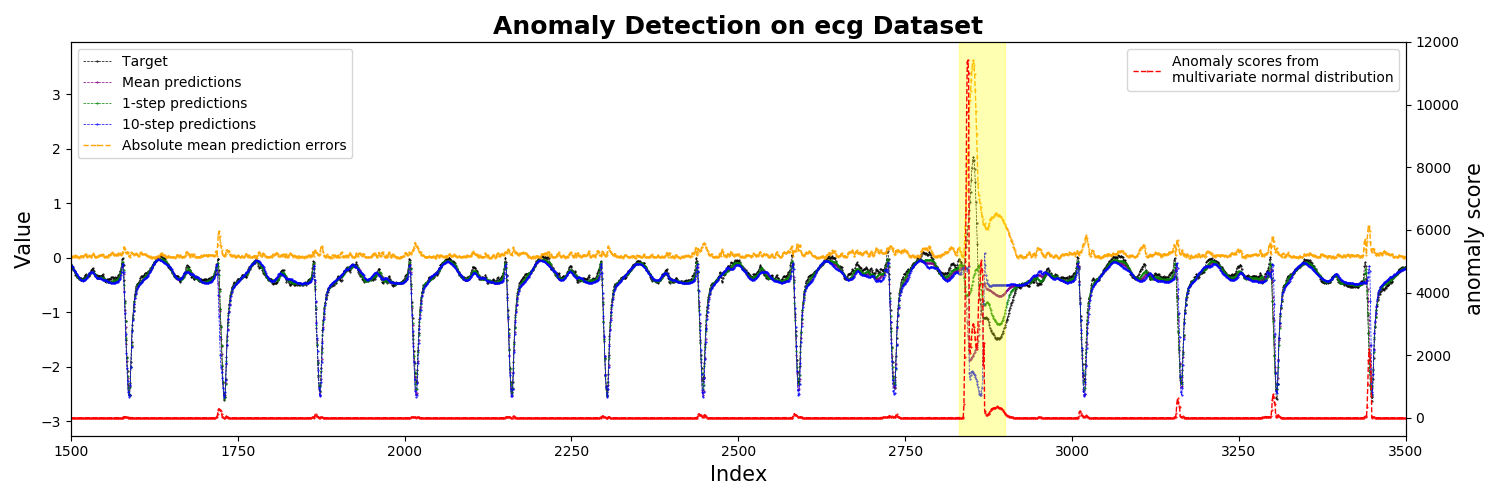## Evaluation

Model performance was evaluated by comparing the model output with the pre-labeled ground-truth. Note that the labels are only used for model evaluation. The anomaly score threshold was increased from 0 to some maximum value to plot the change of precision, recall, and f1 score. Here we show only the results for the ECG dataset. Execute the code yourself and see more results.

1. Precision, recall, and F1 score:

• Electrocardiograms (ECGs) (filename: chfdb_chf14_45590)

a. channel 0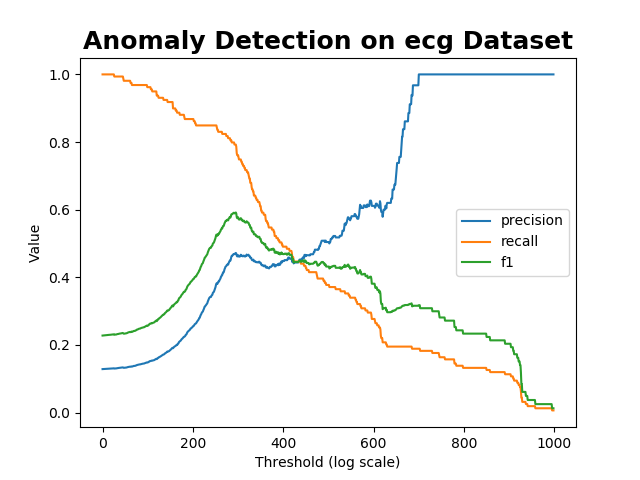b. channel 1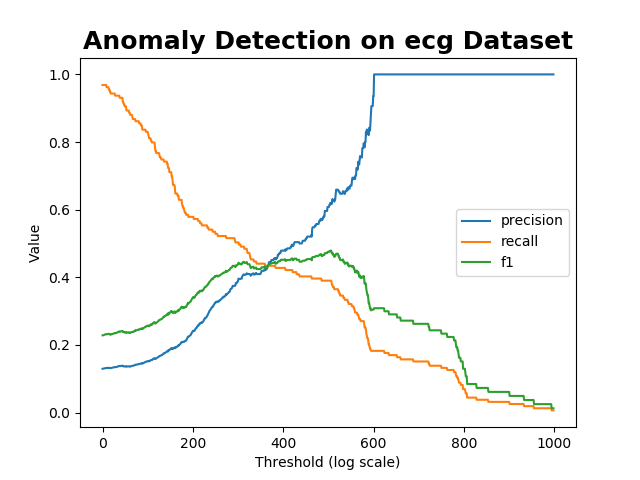## Citations

Get A Weekly Email With Trending Projects For These Topics
No Spam. Unsubscribe easily at any time.
python (53,650
neural-network (735
time-series (237
rnn (166
anomaly-detection (92
prediction (68
forecast (24